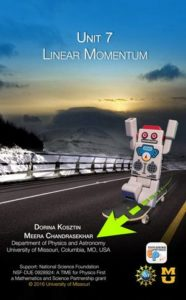### eUnit 7 MomentumBuilding on knowledge from previous units, students study elastic and inelastic collisions using a variety of hands-on activities and labs. Students conduct simple activities to understand the concept of linear momentum and how it is connected to impulse, and to explore different types of collisions. Through qualitative and quantitative labs students investigate the conservation of linear momentum in elastic and inelastic collisions. By the end of this unit students should be able to answer the following questions:

• What is linear momentum?
• How is linear momentum connected to the mass and velocity of an object?
• What is impulse?
• How is impulse connected to linear momentum?
• What is the connection among impulse, forces in a collision and the time of impact?
• How many different types of collisions are there?
• What is the difference between elastic and inelastic collisions?
• What stays the same during a collision?

Suggested timeline for teaching Unit 7

Big Ideas
• Linear momentum describes a relationship between the mass and velocity of an object.
• Linear momentum is conserved in collisions.
• The change in the momentum of an object is called the impulse.
• Impulse represents the average force in a collision multiplied by the time interval of action.
• Linear momentum has both a magnitude and a direction. The direction of linear momentum is the same as that of the velocity.

Learning Goals and Objectives
By the end of this unit, the students should be able to:

1. Investigate various collisions qualitatively by making visual observations. • Compare and contrast an inelastic and an elastic collision.
2. Design an experiment to collect data for two objects in an inelastic collision. • Investigate parameters that affect a collision. • Use a simulation (or technology, such as motion detectors) to conduct an experiment and collect quantitative data on the mass and velocity of objects immediately before and after collisions.
3. Analyze the total linear momentum for an inelastic collision between two objects. • Identify a system in its initial and final states (before and after a collision). • Calculate the linear momentum (p = mv) of an object. • Calculate the initial and final linear momenta of the system. • Compare the initial and final linear momenta of the system. • Describe and apply the law of conservation of momentum. • Manipulate the mass, velocity and direction of motion of colliding objects to see how momentum is conserved in collisions (from data or using a computer simulation).
4. Describe a two-object collision, using multiple representations (i.e., verbal descriptions, pictures, and mathematical models). • Describe in words the conservation of momentum for an inelastic collision. • Draw a picture that indicates the system “before a collision” and “after a collision.” • Apply a mathematical model for the conservation of momentum between two colliding objects.
5. Mathematically determine the linear momentum and change in linear momentum for an object or a system. • Calculate the change in momentum for objects in a collision. • Solve simple collision problems, using the principle of conservation of momentum.
6. Apply the concepts of impulse, force and momentum to collisions and explosions. • Describe the relationship between the force acting on an object in a collision and the change in momentum for that object, FΔt = Δp. • Explain how impulse relates to momentum and force.